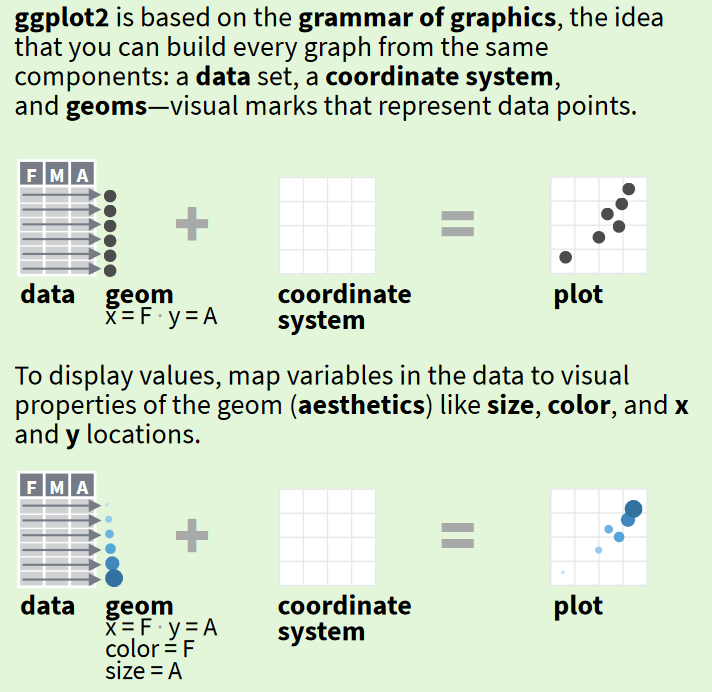# Introduction

This is a lesson introducing you to making plots with the R package ggplot2. The ggplot2 package was originally developed by Hadley Wickham and is now developed and maintained by a huge team of data visualization experts. It’s an elegant and powerful way of visualizing your data and works great for everything from quick exploratory plots to carefully formatted publication-quality graphics.

Students should already have a beginner-level knowledge of R, including basic knowledge of functions and syntax, and awareness of how data frames in R work.

## Learning objectives

At the end of this course, you will know …

• what the “grammar of graphics” is and how ggplot2 uses it
• how to map a variable in a data frame to a graphical element in a plot using `aes`
• how to use different `geom`s to make scatterplots, boxplots, histograms, density plots, and barplots
• how to add trendlines to a plot
• how to compute summary statistics and plot them with `stat` functions
• how to make plots for different subsets of your data using `facet`s
• how to change the style and appearance of your plots

## What is the grammar of graphics?

The theory underlying ggplot2 is the “grammar of graphics.” This concept was originally introduced by Leland Wilkinson in a landmark book. It’s a formal way of mapping variables in a dataset to graphical elements of a plot. For example, you might have a dataset with age, weight, and sex of many individuals. You could make a scatterplot where the age variable in the data maps to the x axis of the dataset, the weight variable maps to the y axis, and the sex variable maps to the color of the points.

In the grammar of graphics, a plot is built in a modular way. We start with data, map variables to visual elements called `geom`s, and then optionally modify the coordinate system and scales like axes and color gradients. We can also modify the visual appearance of the plot in ways that don’t map back to the data, but just make the plot look better.

If that doesn’t make sense to you, read on to see how this is implemented in ggplot2.

## How does a ggplot work?

These images are taken from the ggplot2 cheatsheet. I recommend downloading this cheatsheet and keeping it handy – it’s a great reference!

ggplot2 uses the grammar of graphics to build up all plots from the same set of building blocks. You specify which variables in the data correspond to which visual properties (aesthetics) of the things that are being plotted (geoms).In practice, that looks like this: